##### Trigonometry Workbook For DummiesIf you want to convert from English to metric units or from metric to English units, there is a simple way to do this that uses only one formula for each type of conversion.

## Understand conversion factors

When you multiply any number by 1, that number stays the same. For example, 36 1 = 36. And when a fraction has the same numerator (top number) and denominator (bottom number), that fraction equals 1. So when you multiply a number by a fraction that equals 1, the number stays the same. For example: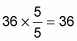If you multiply a measurement by a special fraction that equals 1, you can switch from one unit of measurement to another without changing the value. People call such fractions conversion factors.

Take a look at some equations that show how metric and English units are related (all conversions between English and metric units are approximate):

• 1 meter ≈ 3.26 feet

• 1 kilometer ≈ 0.62 miles

• 1 liter ≈ 0.26 gallons

• 1 kilogram ≈ 2.20 pounds

Because the values on each side of the equations are equal, you can create fractions that are equal to 1, such as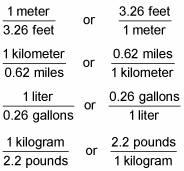After you understand how units of measurement cancel, you can easily choose which fractions to use to switch between units of measurement.

## Cancel units of measurement

When you’re multiplying fractions, you can cancel any factor that appears in both the numerator and denominator. Just as with numbers, you can also cancel out units of measurement in fractions. For example, suppose you want to evaluate this fraction: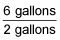You already know that you can cancel out a factor of 2 in both the numerator and denominator. But you can also cancel out the unit gallons in both the numerator and denominator: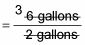So this fraction simplifies as follows:

= 3

## Convert units

After you understand how to cancel out units in fractions and how to set up fractions equal to 1, you have a foolproof system for converting units of measurement.

Suppose you want to convert 7 meters into feet. Using the equation 1 meter = 3.26 feet, you can make a fraction out of the two values as follows: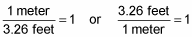Both fractions equal 1 because the numerator and the denominator are equal. So you can multiply the quantity you’re trying to convert (7 meters) by one of these fractions without changing it. Remember that you want the meters unit to cancel out. You already have the word meters in the numerator (to make this clear, place 1 in the denominator), so use the fraction that puts 1 meter in the denominator: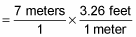Now cancel out the unit that appears in both the numerator and denominator: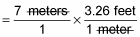At this point, the only value in the denominator is 1, so you can ignore it. And the only unit left is feet, so place it at the end of the expression:

Now do the multiplication:

= 22.82 feet

It may seem strange that the answer appears with the units already attached, but that’s the beauty of this method: When you set up the right expression, the answer just appears.